# Different Types of Chebyshev Filters with Calculations

The name of Chebyshev filters is termed after “Pafnufy Chebyshev” because its mathematical characteristics are derived from his name only. Chebyshev filters are nothing but analog or digital filters. These filters have a steeper roll off & type-1 filter (more pass band ripple) or type-2 filter (stop band ripple) than Butterworth filters. The property of this filter is, it reduces the error between the characteristic of the actual and idealized filter. Because, inherent of the pass band ripple in this filter.

## Chebyshev Filter

Chebyshev filters are used for distinct frequencies of one band from another. They cannot match the windows-sink filter’s performance and they are suitable for many applications. The main feature of Chebyshev filter is their speed, normally faster than the windowed-sinc. Because these filters are carried out by recursion rather than convolution. The designing of the Chebyshev and Windowed-Sinc filters depends on a mathematical technique called as the Z-transform.

## Types of Chebyshev Filters

Chebyshev filters are classified into two types, namely type-I Chebyshev filter and type-II Chebyshev filter.

### Type-I Chebyshev Filters

This type of filter is the basic type of Chebyshev filter. The amplitude or the gain response is an angular frequency function of the nth order of the LPF (low pass filter) is equal to the total value of the transfer function Hn (jw)

Gn(w)=|Hn (jω)|=1√(1+ϵ2Tn2() ω/ωo)

Where,ε = ripple factor
ωo= cutoff frequency
Tn= Chebyshev polynomial of the nth order

The pass-band shows equiripple performance. In this band, the filter interchanges between -1 & 1 so the gain of the filter interchanges between max at G = 1 and min at G =1/√(1+ε2) . At the cutoff frequency, the gain has the value of 1/√(1+ε2) and remains to fail into the stop band as the frequency increases. The behavior of the filter is shown below. The cutoff frequency at -3dB is generally not applied to Chebyshev filters.

The order of this filter is similar to the no. of reactive components required for the Chebyshev filter using analog devices. The ripple in dB is 20log10 √(1+ε2). So that the amplitude of a ripple of a 3db result from ε=1 An even steeper roll-off can be found if ripple is permitted in the stop band, by permitting 0’s on the jw-axis in the complex plane. Though, this effect in less suppression in the stop band. The effect is called a Cauer or elliptic filter.

#### Poles and Zeros of Type-I Chebyshev Filter

The poles and zeros of the type-1 Chebyshev filter is discussed below. The poles of the Chebyshev filter can be determined by the gain of the filter.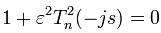-js=cos(θ) & the definition of trigonometric of the filter can be written as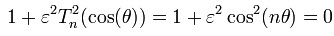Here θ can be solved by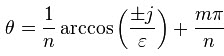Where the many values of the arc cosine function have made clear using the number index m. Then the Chebyshev gain poles functions are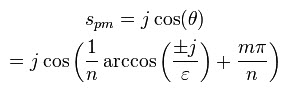Using the properties of hyperbolic & the trigonometric functions, this may be written in the following form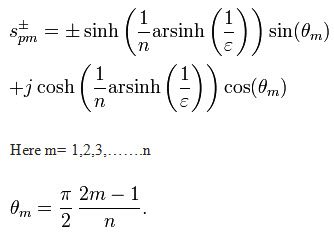The above equation produces the poles of the gain G. For each pole, there is the complex conjugate, & for each and every pair of conjugate there are two more negatives of the pair. The TF should be stable, The transfer function (TF) is given by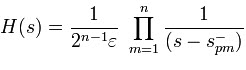### Type-II Chebyshev Filter

The type II Chebyshev filter is also known as an inverse filter, this type of filter is less common. Because, it doesn’t roll off and needs various components. It has no ripple in the passband, but it has equiripple in the stopband. The gain of the type II Chebyshev filter is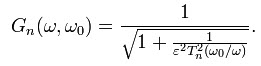In the stopband, the Chebyshev polynomial interchanges between -1& and 1 so that the gain ‘G’ will interchange between zero and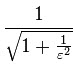The smallest frequency at which this max is reached is the cutoff frequency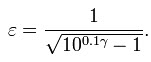For a 5 dB stop band attenuation, the value of the ε is 0.6801 and for a 10dB stop band attenuation the value of the ε is 0.3333. The cutoff frequency is f0 = ω0/2π0 and the 3dB frequency fH is derived as

####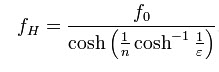Poles and Zeros of Type-II Chebyshev Filter

Assume the cutoff frequency is equal to 1, the poles of the filter are the zeros of the gain’s denominator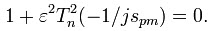The poles of the gain of  type II filter are the opposite of the poles of the type I Chebyshev filter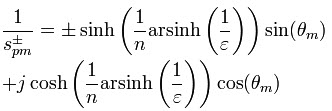Here in the above equation m = 1, 2, …, n. The zeroes of the type II filter are the zeroes of the gain’s numerator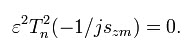The zeroes of the type II Chebyshev filter are opposite to the zeroes of the Chebyshev polynomial.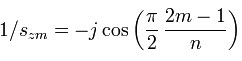Here, m = 1,2,3,………n

By using a left half plane, the TF is given of the gain function and has the similar zeroes which are single rather than dual zeroes.

Thus, this is all about Chebyshev filter, types of Chebyshev filter, poles and zeros of Chebyshev filter and transfer function calculation. We hope that you have got a better understanding of this concept, furthermore any queries regarding this topic or electronics projects, please give your feedback by commenting in the comment section below. Here is a question for you, what are the applications of Chebyshev filters?